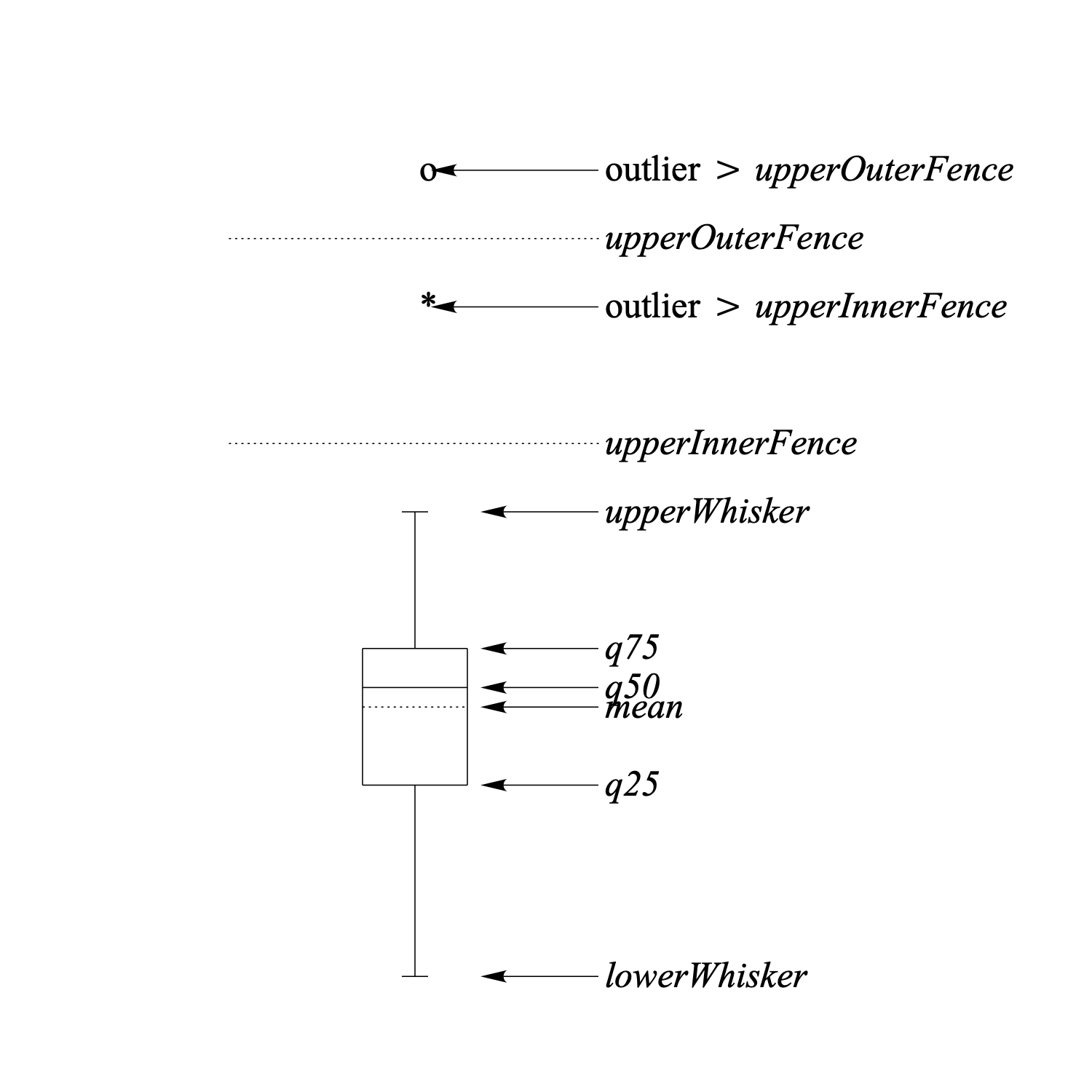box plot

A box plot provides a simple graphical summary of data. These plots originate from the work of Tukey (1977).

### Definitions

The following figure shows an annotated box plot.To understand the box plot we need the following definitions:

q25 = lower quartile, 25% of the data lie below this value
q50 = median, 50% of the data lie below this value
q75 = upper quartile, 25% of the data lie above this value

The following definitions all depend on these quantiles:

hspread = |q75q25| (50% interval)
lowerOuterFence = q25 – 3.0 * hspread (not in figure)
lowerInnerFence = q25 – 1.5 * hspread (not in figure)
upperInnerFence = q75 + 1.5 * hspread
upperOuterFence = q75 + 3.0 * hspread
lowerWhisker = smallest data value larger then lowerInnerFence
upperWhisker = largest data value smaller then upperInnerFence

The box plot is a summary of the data in which:

•  the horizontal lines of the rectangle correspond to q25, q50 and q75, respectively.
•  the dotted line corresponds to the mean.
•  the outliers outside the outerFences are drawn with an 'o'.
•  the outliers in the intervals (lowerOuterFence, lowerInnerFence) and (upperInnerFence, upperOuterFence) are drawn with an '*'.
•  the whisker lines outside the rectangle connect q25 with lowerWhisker, and, q75 with upperWhisker, respectively. With no outliers present, the whiskers mark minimum and/or maximum of the data.